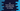# C# program to find the maximum occurring character in a string## C# program to find the maximum occurring character in a string:

In this post, we will learn how to find the maximum occurring character in a string. For that, we will take the string  as input from the user. The program will find the maximum occurring character in that string and print it out.

If we have more than one character with an equal number of occurrences, then it will print the character that comes first in alphabetical order.

For example, if the string is hello world, it will print l, which is occurring 3 times in this string.

## Algorithm to find the maximum occurring character in a string:

To find the maximum occurring character in a string in C#, we will use the below algorithm:

• Read the string as input from the user.
• Initialize one array of size 256. Initialize each item in the array as 0.  We are initializing this array to hold the total count of each character in that string. Each index in the array defines the ASCII value of a character.
• Read each character of the string one by one. For each character’s ASCII value, increment the value in the array by 1. For example, if the character is a, increment the value in the array at index 97, because 97 is the ASCII value of a.
• Once the loop will end, we will have to iterate through the array to find the index with maximum value.This index is the ascii of the character that occurs maximum time in that string. Print the character for that ASCII value.

### C# program:

Below is the complete program:

``````using System;
namespace Main {
public class Example {

private static char getMaxOccurrenceCharacter(String str) {
int[] countArray = new int;
int maxValue = 0;
char resultChar = '\0';

for (int i = 0; i < str.Length; i++) {
countArray[str[i]]++;

if (countArray[str[i]] > maxValue) {
maxValue = countArray[str[i]];
resultChar = str[i];
}
}

return resultChar;
}

public static void Main() {
Console.WriteLine("Enter a string :");

Console.WriteLine("The character with maximum occurrence is : " + getMaxOccurrenceCharacter(givenStr));

}
}
}``````

Here,

• getMaxOccurrenceCharacter method is used to get the character with maximum occurrence in a string. str is the provided string.
• countArray is the array to hold the count of each character.
• maxValue is used to hold the maximum value found in the array and resultChar  to hold the final character.
• We are using only one for loop here. This loop runs through the characters of the string and updates the countArray members. Inside the loop, we are also finding the current maximum value in the array and current character with max number of occurrence.
• This method retuns the resultChar

If you run this program, it will print output as like below:

``````Enter a string :
hello worldddd

The character with maximum occurrence is : d``````